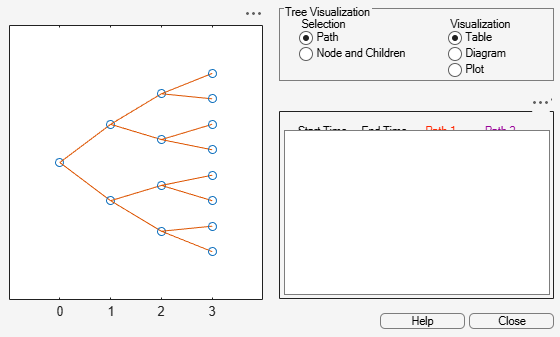# bushshape

Retrieve shape of bushy tree

## Syntax

``[NumLevels,NumChild,NumPos,NumStates,Trim] = bushshape(Tree)``

## Description

example

````[NumLevels,NumChild,NumPos,NumStates,Trim] = bushshape(Tree)` returns information on a bushy tree's shape. ```

## Examples

collapse all

`load deriv.mat; `

With `treeviewer` you can see the general shape of the HJM interest-rate tree.

`treeviewer(HJMTree)`Use `bushshape` with the `HJMTree`.

`[NumLevels, NumChild, NumPos, NumStates, Trim] = bushshape(HJMTree.FwdTree) `
```NumLevels = 4 ```
```NumChild = 1×4 2 2 2 0 ```
```NumPos = 1×4 4 3 2 1 ```
```NumStates = 1×4 1 2 4 8 ```
```Trim = logical 1 ```

You can recreate this tree using the `mkbush` function.

`Tree = mkbush(NumLevels, NumChild(1), NumPos(1), Trim)`
```Tree=1×4 cell array {4x1 double} {3x1x2 double} {2x2x2 double} {1x4x2 double} ```
`Tree = mkbush(NumLevels, NumChild, NumPos)`
```Tree=1×4 cell array {4x1 double} {3x1x2 double} {2x2x2 double} {1x4x2 double} ```

## Input Arguments

collapse all

Bushy tree, specified using an HJM, BDT, HW, BK, or CIR tree.

Data Types: `struct`

## Output Arguments

collapse all

Number of tree levels, returned as a numeric.

Number of branches (children) of the nodes in each level, returned as a `1`-by-number of levels (`NUMLEVELS`) vector.

Length of the state vectors in each level, returned as a `1`-by-number of levels (`NUMLEVELS`) vector.

Number of state vectors in each levels, returned as a `1`-by-number of levels (`NUMLEVELS`) vector.

Trim, returned as a `1` if `NumPos` decreases by `1` when moving from one time level to the next. Otherwise, it is `0`.